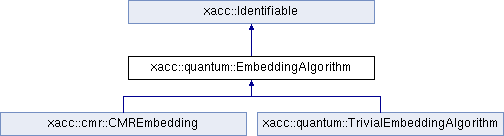XACC
xacc::quantum::EmbeddingAlgorithm Class Referenceabstract

`#include <EmbeddingAlgorithm.hpp>`

Inheritance diagram for xacc::quantum::EmbeddingAlgorithm:## Public Member Functions

EmbeddingAlgorithm ()

virtual ~EmbeddingAlgorithm ()

virtual Embedding embed (std::shared_ptr< Graph > problem, std::shared_ptr< Graph > hardware, std::map< std::string, std::string > params=std::map< std::string, std::string >())=0Public Member Functions inherited from xacc::Identifiable
virtual const std::string name () const =0

virtual const std::string description () const =0

virtual ~Identifiable ()

## Detailed Description

The EmbeddingAlgorithm class provides an interface for minor graph embedding algorithms.

## Constructor & Destructor Documentation

 xacc::quantum::EmbeddingAlgorithm::EmbeddingAlgorithm ( )
inline

The Constructor

 virtual xacc::quantum::EmbeddingAlgorithm::~EmbeddingAlgorithm ( )
inlinevirtual

The Destructor

## Member Function Documentation

 virtual Embedding xacc::quantum::EmbeddingAlgorithm::embed ( std::shared_ptr< Graph > problem, std::shared_ptr< Graph > hardware, std::map< std::string, std::string > params = `std::map< std::string, std::string >()` )
pure virtual

Implementations of EmbeddingAlgorithm implement this method to provide a valid minor graph embedding of the given problem graph into the given hardware graph.

Parameters
 problem The problem graph to be embedded into the hardware graph hardware The hardware graph. params Any key-value string parameters to influence the algorithm.
Returns
embedding A mapping of problem vertex indices to the list of hardware vertices they map to

Implemented in xacc::quantum::TrivialEmbeddingAlgorithm.

The documentation for this class was generated from the following file: